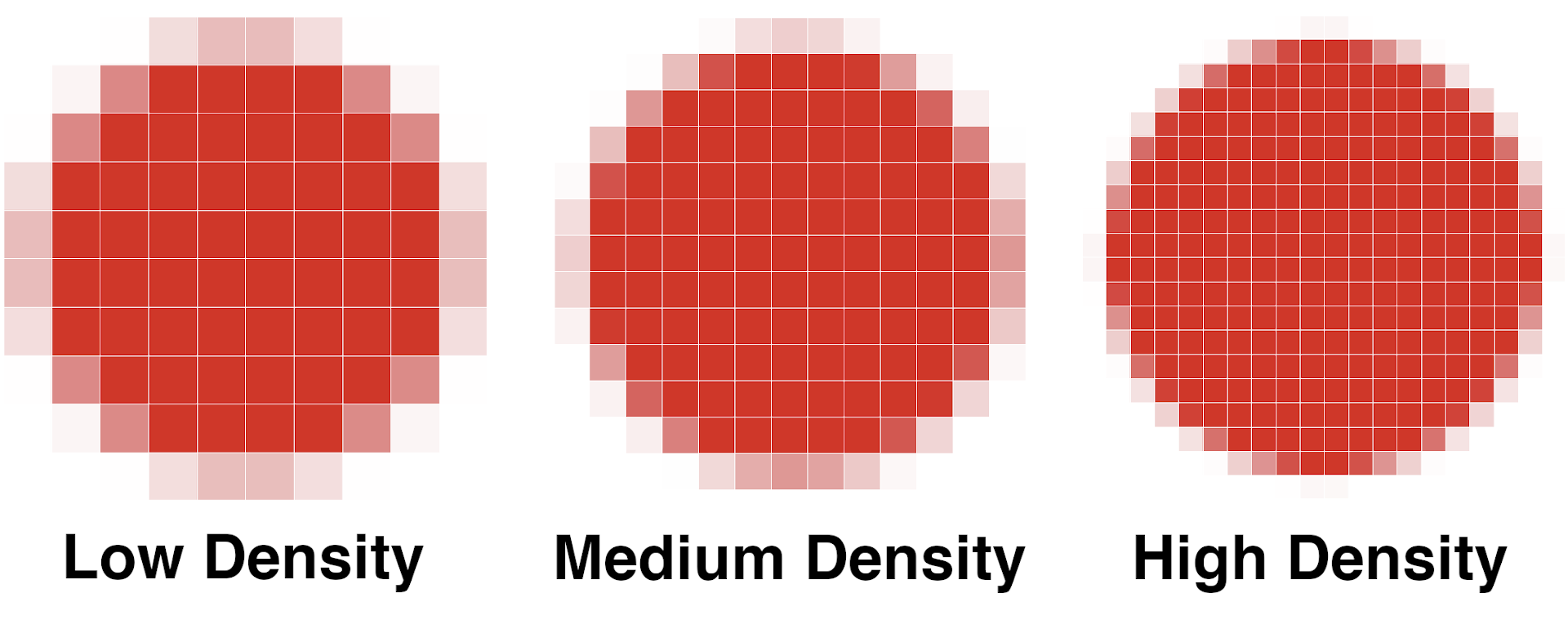# PPI Calculator / DPI Calculator

Calculate the pixels per inch, or PPI, or a screen given the diagonal size and aspect ratio using the calculator below.

Screen Resolution
:
Enter the screen's width in pixels by the height in pixels

## PPI/DPI Results:

PPI: 91.79 8,425 2,203 0.2767 2,073,6002.074 MP 16:9
Learn how we calculated this below

## How to Calculate PPI

Pixels per inch, abbreviated PPI, is a measure of the pixel density of a screen or display. This is different from the display resolution as it’s the proportion of the number of pixels to the display size.There are three steps to calculating a screen’s PPI.

### Step One: Find the Screen’s Diagonal

The first step to calculate PPI is to measure the screen’s diagonal size in inches. Most displays, screens, monitors, and televisions are sold by their diagonal measurement, which should be marked on the screen or its documentation.

If not, measure the diagonal from one corner to the opposite corner using a tape measure. Or, simply use our screen size calculator to find the size.

### Step Two: Find the Diagonal Pixels

The second step to calculating PPI is finding the total number of pixels along the diagonal measurement. This might seem complicated, but you can use the Pythagorean theorem to find this using the screen’s resolution.

Given the screen’s resolution, you can find the number of pixels along the diagonal using the Pythagorean theorem.

dp = w2 + h2

In other words, the diagonal pixels dp is equal to the square root of the width in pixels w squared plus the height in pixels h squared.

The width in pixels is equal to the first part of the screen’s resolution, and the height is the second. For instance, the width in pixels of a 1920×1080 screen is 1920, and the height is 1080.

### Step Three: Use the PPI Formula

Given the diagonal measurement in pixels and inches, use the following formula to calculate PPI.

PPI = dp / di

Thus, screen pixel density in pixels per inch is equal to the pixels along the diagonal dp divided by the diagonal in inches di.

## PPI vs. DPI – Are They the Same?

Dots per inch, or DPI, are very similar to pixels per inch, but they are slightly different. While pixels per inch is a measure of screen density in pixels, dots per inch is a measure of the density in dots.

Dots, sometimes also called points when referring to a screen, might encompass multiple pixels or might be smaller than a pixel depending on the resolution and pixel size.

DPI is most often used when measuring the resolution of a printer, which prints in dots rather than 1:1 to the pixels of the screen. DPI is also used when referring to the computer mouse sensitivity and how far the mouse cursor will move on the screen for a given distance of actual movement.

## What is Retina?

Retina is a brand name trademarked by apple and refers to a display with a pixel density such that the individual pixels are discernible to the human eye.

The actual density will vary depending on how far your eyes are generally from the screen, but a PPI of 300 is generally enough density when viewing from 12″ away.

## What is a Good PPI Value?

At this point, you might be wondering what a good screen density value might be? A “good” PPI density will vary depending on the use of the screen and the individual user.

For example, a computer monitor viewed very closely might require a higher pixel density than a small TV. However, the industry has some agreement on what is considered low, medium, and high pixel densities.

### Pixel Density Categories

The table below shows the defined categories of display pixel densities.

Standard screen pixel density categories
Abbreviation Name DPI Range
LDPI low-density 0-120 DPI
MDPI medium-density 120-160 DPI
TVDPI medium-high-density 160-213 DPI
HDPI high-density 213-240 DPI
XHDPI extra-high-density 240-320 DPI
XXHDPI extra-extra-high-density 320-480 DPI
XXXHDPI extra-extra-extra-high-density 480-640 DPI

If you’re looking for the right viewing distance for a display given it’s resolution, try our TV size and distance calculator.

## References

1. Android Developers, App resources overview, https://developer.android.com/guide/topics/resources/providing-resources#AlternativeResources